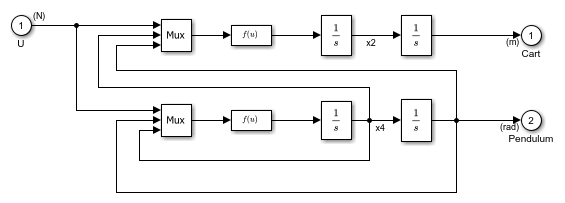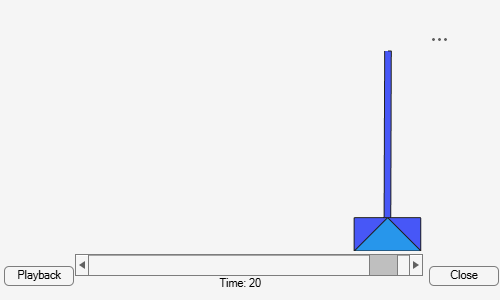# Inverted Pendulum with Animation

This example shows how to use a Simulink® to model and animate an inverted pendulum system. An inverted pendulum has its center of mass above its pivot point. To stably maintain this position, the system implements control logic to move the pivot point below the center-of mass as the pendulum starts to fall. The inverted pendulum is a classic dynamics problem used to test control strategies.In this example, the system consists of an inverted pendulum mounted to a mobile cart. The model constrains the motion to the vertical plane. To maintain the system stability, the model implements state feedback control to track the cart position.### Animation

The model uses a Level-2 MATLAB S-Function block to implement the animation. The S-function uses MATLAB® Handle Graphics®. To view the S-function, open `pendan.m`.### Inverted Pendulum Dynamics

The model uses a masked subsystem to implement the inverted pendulum nonlinear system dynamics. The subsystem determines the cart position along the x -axis and the pendulum rotation about the pivot point.### State Feedback Control

The model implements state feedback control to track the position of the cart and maintain the pivot point below the pendulum center of mass. The `State Estimator` subsystem uses a Luenberger observer model to estimate the internal states of the system.

### Analysis

The Scope block provides signal output as the simulation runs. You can also use the Simulation Data Inspector to investigate the logged signals.